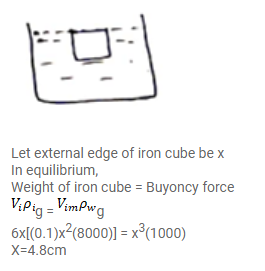# A cubical box is to be constructed with iron sheets

Question:

A cubical box is to be constructed with iron sheets $1 \mathrm{~mm}$ in thickness. What can be the minimum value of the external edge so that the cube does not sink ink water?

Density of iron $=8000 \mathrm{~kg} / \mathrm{m}^{3}$ and density of water $=1000 \mathrm{~kg} / \mathrm{m}^{3}$

Solution: